Isn’t predicting the future the dream come true or the biggest magic for everyone? The data we have at this time can be used to get more than a peek into the future. Time series forecasting gives us the power to predict future observations and is extremely useful in stock market analysis, product demand analysis, and so on.“ With great power comes great responsibility.’’ so, let’s dive in and learn more.

You may think this is just like regression, we already have some examples known, we are just predicting value for new examples.nut the challenge is that observations are time-dependent here and usually have seasonal trends.

Time-series:   series of data points collected over time with time stamps.

The data depends upon a time as shown below (price of houses Vs year)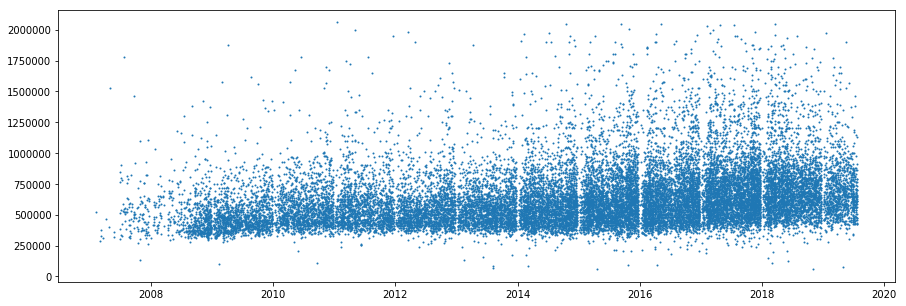We see an increasing trend in house prices with the year.

The main components of a time series are:

Trend: depicts how the value is changing over time increasing or decreasing.

Seasonality: in some time-dependent problems, there is a sudden peak or low during a certain period. for example, watermelon, AC sales will be high during summer every year. by plotting or visualization, we can observe the seasonality trends which are impactful.

Noise: unnecessary disturbances

Before applying the time series forecasting, we need to make sure that the time series is stationary.

What is the meaning of stationary time series?

• The mean of the series should be constant
• The variance or standard deviation of the series should not vary with time

Only if a time series is stationary, we can do better forecasting. we can easily apply mathematical formulas and models.

How to check if a time series is stationary?

• Rolling statistics:

You can plot the rolling mean and standard deviation and check if it is a straight line.

• Augmented Dickey-Fuller Test:

The time series is considered stationary if the p-value is low and the test statistics should be less than the critical values.

We’ll be using the below dataset as a reference to learn how to implement time series forecasting.(choose the Electric_Production.csv)

https://www.kaggle.com/shenba/time-series-datasets

As you can see, the dataset has 2 columns, timestamp, and the amount of electricity production. Let us see how to preprocess this dataset.

First, we need to preprocess the dataset and visualize it.

Import numpy, pandas,matplotlib like usually.

Statsmodel library is imported, as it is used for dealing with time-series data.

Read the dataset and display it.

Python

Let us visualize the data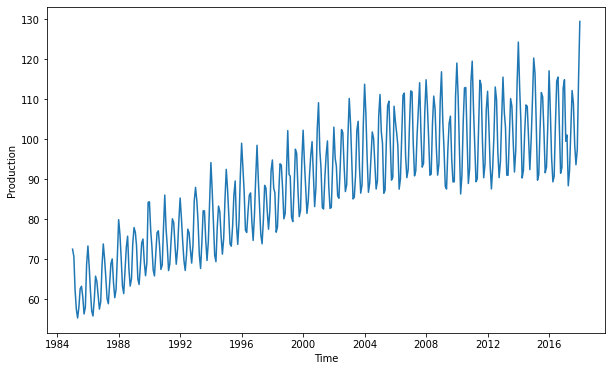Now, its clearly visible that we have an increasing trend. To check if it is stationary, we need to plot the rolling statistics of time series.

Rolling mean also called as Moving Average is calculated to see the long term picture avoiding the short fluctuations.click on the link to learn more if you are not familiar with statistics.

Pandas allow you to compute rolling mean using .rolling().mean() and standard deviation using .rolling.std() methods

Note: we set the argument window=12,(for 12 months) ,to get an rolling mean annually.

Python

Now ,to check if the time series is stationary,we plot the rolling average and rolling standard deviation.

Python

From the output plot below, we can see that both conditions of stationarity are not satisfied. Even though the rolling standard deviation is not varying with time, the rolling mean is increasing with time.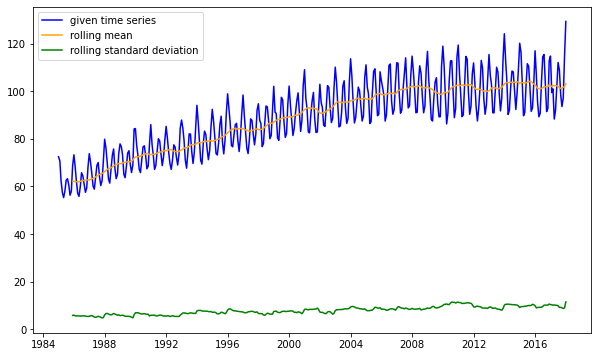How to make the time series stationary?

We want to make rolling mean constant. for that, we need to step by step to reduce the rate at which it’s increasing.

• Log scale transformation
• Exponential decay
• Time shift transformation.

First, we’ll execute the log scale transformation method.

One of the simple ways for the same is to take log of rolling mean

Python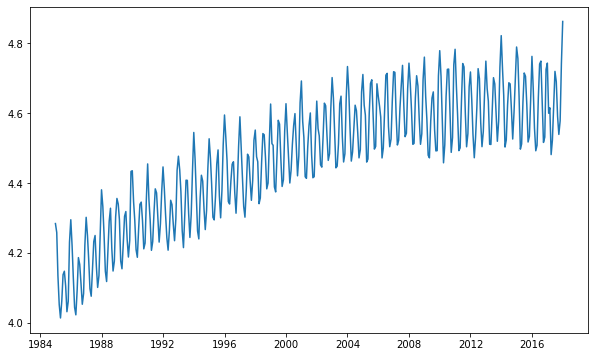This is the transformed new data plot.

To make the rolling mean independent .

Python

We have calculated the rolling mean of the transformed time series, stored it in new_rolling_mean. Now, we subtract this from all values in the dataset, in order to make rolling mean constant..

Python

Now lets plot and ensured that the transformed time series is stationary.

Python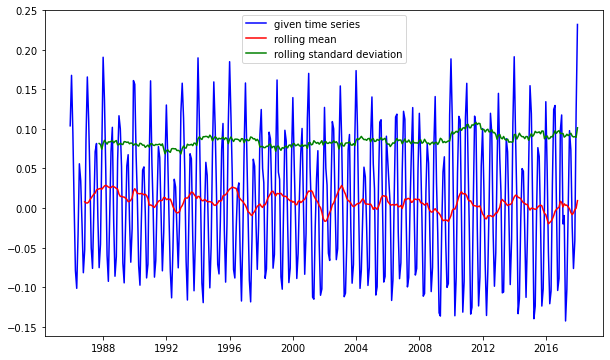As you can see, both rolling mean and rolling standard deviation are independent over time .the time series is now stationary.

Now, we can apply all statistical models.

ARIMA – AutoRegressive Integrated Moving Average Model

AR (auto regression) – this follows the principle of linear regression, predicts future values as a function of the previous year’s values.

Order of Autoregressive model is no of the preceding values that we are going to use to predict the present value.

MA(Moving Average)- this is a model that proposes that the current values can be predicted as a linear regression of previous errors.

The order of Moving Average Model denotes the no of moving average terms.

ARIMA is a model that combines both autoregressive and moving average model, along with differencing (in the case on non-stationary)

The three main parameters of the ARIMA model are:

• d: Differencing Order

If a series is not stationary, we do differencing to make it stationary.

(i.e, we find  current time period – previous time period)

We’ll have to do differencing as many times till the time series become stationary.so, d is the minimum number of differencing transformations required.

• p: AR(Auto-Regressive) Order

This is the order of the AR model or no of lags used in the prediction.

• q: MA(Moving Average) Order

This denotes the MA order or no of moving average terms. It is       nothing  but the no of lagged errors we will be using

For an arima model if q=0, then it is purely an Autoregressive model.

Similarly, if p=0, then it is purely a Moving Average ModelWe need to find out p and q parameters to implement ARIMA.

HOW TO DECIDE THE PARAMETERS OF ARIMA?

For determining ‘d’, we can perform the differencing and plot to check if time series has become stationary. So, we’ll know how many times is the minimum required.along with plotting, ADFC test will also help in this.

For determining ‘q’ or order of the moving average, we need to perform ACF.

Auto Correlation Function (ACF)

In this, we correlate between the current values and previous values at all times i.e, to visualize the correlation of time series throughout.

For the differenced series(stationary), we will have to plot ACF to find out q.

Partial Auto Correlation Function (PACF)

For determining ‘p’ or order of the AutoRegressive, we need to perform PACF.

This allows us to correlate a time series to a particular lag when we do not consider the influence or impact of all other lags in between.

Going back to the problem we were working with , let us try to plot PACF and ACF for the same and hence use ARIMA on it.

from statsmodels.tsa.stattools import acf, pacf

This allows you to use acf() and pacf() functions to plot .

( you can refer the complete code in Google collab notebook)

From the plot, our ‘p’ will be the x-value at which the autocorrelation becomes zero (intersects the purple line)  in the ACF plot. From the below plot, you can see that “p=2”
Similarly, our  ‘q’ will be the X-value at which partial autocorrelation becomes zero(intersects the purple line) in the PACF plot. from the below plot, we can see that “q=0”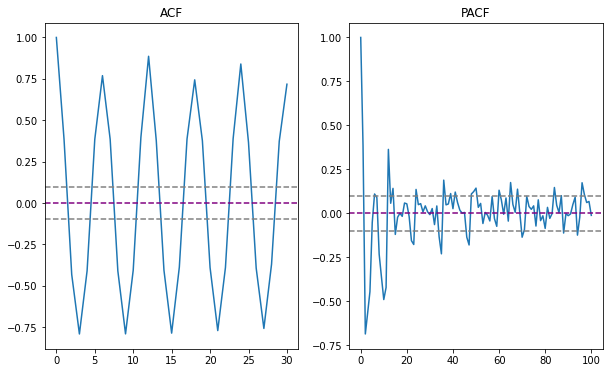From earlier, we got a stationary time series after one transformation.

So, d=2,

Hence, for the problem in case parameters are d=1,p=2,and q=2.

From statsmodel library, we import arima

from statsmodels.tsa.arima_model import ARIMA

order =(p,d,q)

[ AR + I + MA ]

So, model = ARIMA(data_log, order=(2,1,2)

The arima model is fitted as shown.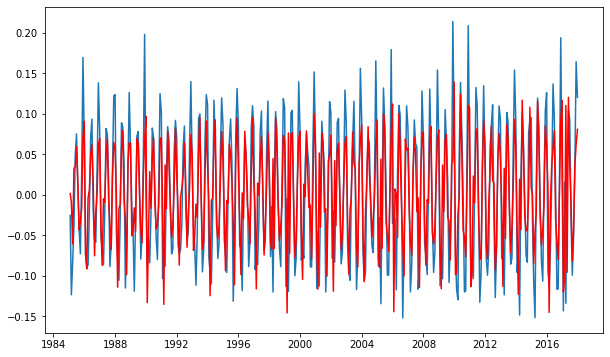Now we can make predictions using the model. Then, as all this is done on log-transformed data, we need to do reverse transformation and go back to the original form.

Note: we did np.log() during log transformation, so to reverse it now we will do np.exp()

(refer to code )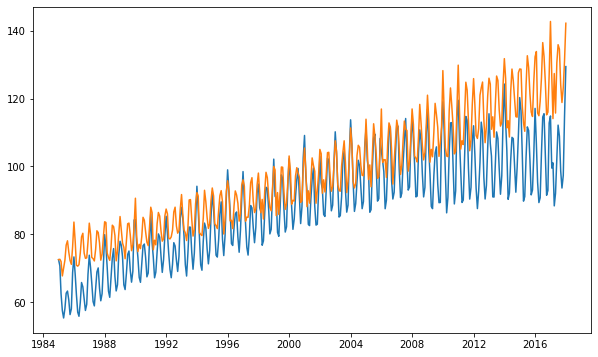Using plot_predict(), you can forecast and visualize as shown below…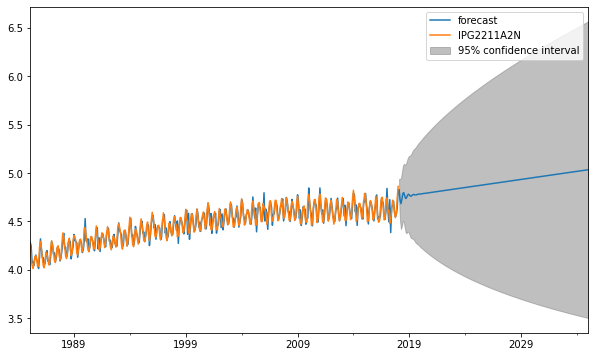So, you should be able to paint a clear picture of the basics of time series forecasting and ARIMA model by now. In cases, where there is seasonality too in the time series, we go for more advanced models like SARIMA.

I hope you understood the concept and implementation of Time Series. If you have any suggestions, drop it in the comments